# Circuit Theorems – 1 – 2

6. Thevenin’s theorem can be applied to …….

(a) d.c. circuits only
(b) a.c. circuits only
(c) both d.c. and a.c. circuits
(d) none of these

Explanation
No answer description available for this question. Let us discuss.

7. Below given symbol represents :(a) voltage source
(b) current source
(c) capacitor
(d) resistor

Explanation
No answer description available for this question. Let us discuss.

8. Below given symbol represents :(a) voltage source
(b) current source
(c) capacitor
(d) resistor

Explanation
No answer description available for this question. Let us discuss.

9. An ideal voltage source should have :

(a) zero internal resistance
(b) infinite internal resistance
(c) terminal voltage in proportion to current
(d) terminal voltage in proportion to load

Explanation
No answer description available for this question. Let us discuss.

10. An ideal current source should have :

(a) zero internal resistance
(b) infinite internal resistance
(c) terminal voltage in proportion to current
(d) terminal voltage in proportion to load

Explanation
No answer description available for this question. Let us discuss.

General Knowledge Books##General Knowledge 2019 Price: INR 30.00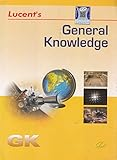-17% General Knowledge Price: INR 179.00 Was: INR 216.00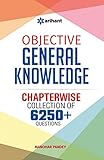-43% Objective General Knowledge Chapterwise Collection of 6250+ Questions Price: INR 169.00 Was: INR 295.00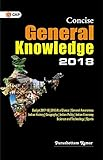Concise General Knowledge 2018 Price: INR 30.00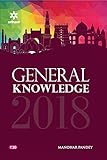General Knowledge 2018 (Old Edition) Price: Check on Amazon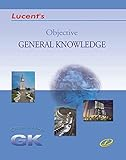-16% Objective General Knowledge Price: INR 302.00 Was: INR 360.00 ‹›

Be a Part of the New & Next

Share the Knowledge

Copy Protected by Chetan's WP-Copyprotect.